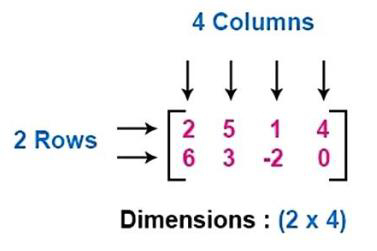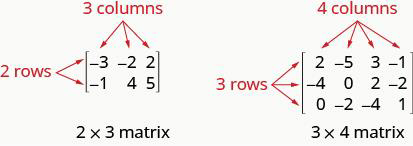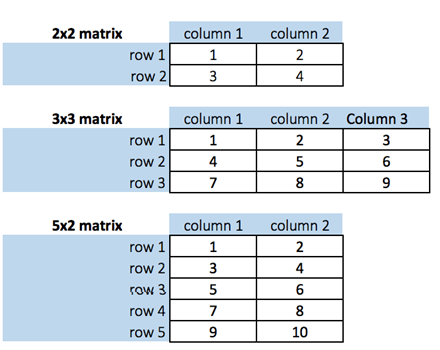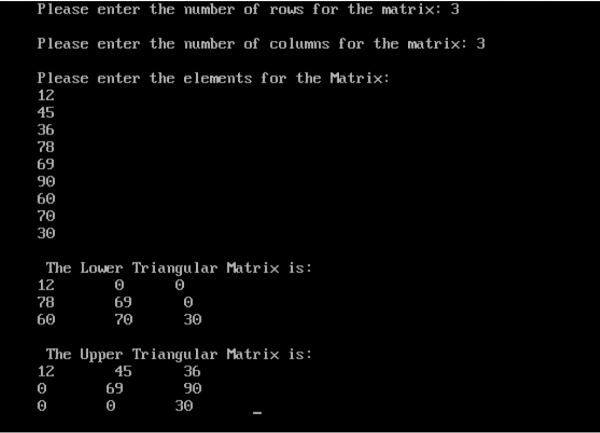# Program to print Lower triangular and Upper triangular matrix of an array in C

CServer Side ProgrammingProgramming

## Program Description

Write a program to print lower triangular matrix and upper triangular matrix of an Array.

Triangular Matrix

A Triangular matrix is one that is either lower triangular or upper triangular.

Lower Triangular Matrix

A square matrix is called lower triangular if all the entries above the main diagonal are zero.

Upper Triangular Matrix

A square matrix is called upper triangular if all the entries below the main diagonal are zero.

A matrix of the form

$$L={\begin{bmatrix}\ell _{1,1}&&&&0\\\ell _{2,1}&\ell _{2,2}&&&\\\ell _{3,1}&\ell _{3,2}&\ddots &&\\\vdots &\vdots &\ddots &\ddots &\\\ell _{n,1}&\ell _{n,2}&\ldots &\ell _{n,n-1}&\ell _{n,n}\end{bmatrix}}$$

is called a lower triangular matrix or left triangular matrix, and analogously a matrix of the form

$$U={\begin{bmatrix}u_{1,1}&u_{1,2}&u_{1,3}&\ldots &u_{1,n}\\&u_{2,2}&u_{2,3}&\ldots &u_{2,n}\\&&\ddots &\ddots &\vdots \\&&&\ddots &u_{n-1,n}\\0&&&&u_{n,n}\end{bmatrix}}$$

is called an upper triangular matrix or right triangular matrix. A lower or left triangular matrix is commonly denoted with the variable L, and an upper or right triangular matrix is commonly denoted with the variable U or R.

A matrix that is both upper and lower triangular is diagonal. Matrices that are similar to triangular matrices are called triangularisable.

Example − Upper Triangular Matrix

$${\begin{bmatrix}{1}&{4}&{1}\\{0}&{6}&{4}\\{0}&{0}&{1}\end{bmatrix}}$$

Example − Lower Triangular Matrix

$${\begin{bmatrix}{1}&{0}&{0}\\{2}&{8}&{0}\\{4}&{9}&{7}\end{bmatrix}}$$

## Algorithm

Examples − Different Dimensions of MatricesFor lower triangular matrix

Find the Index position of row and column.

If column position is greater than row position, then make that position 0.

For upper triangular matrix

Find the index position of row and column.

If column position is smaller than row position then make that position 0.## Example

/* Program to find Lower and Upper Triangle Matrix */
#include<stdio.h>
int main() {
int rows, cols, r, c, matrix;
clrscr(); /*Clears the Screen*/
printf("Please enter the number of rows for the matrix: ");
scanf("%d", &rows);
printf("\n");
printf("Please enter the number of columns for the matrix: ");
scanf("%d", &cols);
printf("\n");
printf("Please enter the elements for the Matrix: \n");
for(r = 0; r < rows; r++){
for(c = 0;c < cols;c++){
scanf("%d", &matrix[r][c]);
}
}
printf("\n The Lower Triangular Matrix is: ");
for(r = 0; r < rows; r++){
printf("\n");
for(c = 0; c < cols; c++){
if(r >= c){
printf("%d\t ", matrix[r][c]);
}
else{
printf("0");
printf("\t");
}
}
}
printf("\n\n The Upper Triangular Matrix is: ");
for(r = 0; r < rows; r++){
printf("\n");
for(c = 0; c < cols; c++){
if(r > c){
printf("0");
printf("\t");
}
else{
printf("%d\t ", matrix[r][c]);

}
}
}
getch();
return 0;
}

## Output